Introduction to Electronic Circuits: 1 of 3

The electronic devices we encounter all around us are driven and controlled by the flow of electrical current through electronic circuits. Each circuit is an arrangement of electrical elements designed to perform specific functions. Circuits can be engineered to carry out a wide variety of operations, from simple actions to complex tasks, according to the job(s) the system must perform.

Let's begin by looking at how the key passive elements found in most electronic circuits work.

A passive element is an electrical component that does not generate power, but instead dissipates, stores, and/or releases it. Passive elements include resistances, capacitors, and coils (also called inductors). These components are labeled in circuit diagrams as Rs, Cs and Ls, respectively. In most circuits, they are connected to active elements, typically semiconductor devices such as amplifiers and digital logic chips.

## Resistors

A resistor is a primary type of physical component that is used in electronic circuits. It has two (interchangeable) leads. The material placed internally between the two leads of a resistor opposes (restricts) the flow of current. The amount of that opposition is called its resistance, which is measured in ohms (Ω). Resistors are used to control the various currents in areas of a circuit and to manage voltage levels at different points therein by producing voltage drops. When a voltage is applied across a resistor, current flows through it. Ohm's law for resistors is E = IR, where E is the voltage across the resistor, R is the resistance of the resistor, and I is the current flowing through the resistor. That current is proportional to the applied voltage, and inversely proportional to the resistance. Thus, as resistance goes up, the current through the element comes down, so that at high resistances the current is very small.

Ohm's law makes it possible to calculate any one of three circuit values (current, voltage, or resistance) from the other two.

## Capacitors

A capacitor is another primary type of physical component used in electronic circuits. It has two leads and is used to store and release electric charge. A capacitor's ability to store charge is referred to as its capacitance, measured in farads (F).

A typical capacitor takes the form of two conductive plates separated by an insulator (dielectric). This type of circuit element cannot pass direct current (DC) because electrons cannot flow through the dielectric. However, a capacitor does pass alternating current (AC) because an alternating voltage causes the capacitor to repeatedly charge and discharge, storing and releasing energy. Indeed, one of the major uses of capacitors is to pass alternating current while blocking direct current, a function called 'AC coupling'.

When a direct current flows into a capacitor, a positive charge rapidly builds up on the positive plate and a corresponding negative charge fills the negative plate (see Figure 1). The buildup continues until the capacitor is fully charged—i.e., when the plates have accumulated as much charge (Q) as they can hold. This amount is determined by the capacitance value (C) and the voltage applied across the component: (Q = CV). At that point, current stops flowing (see Figure 2).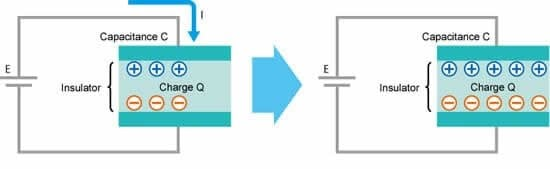Figure 1: The capacitor is charging / Figure 2: The capacitor is charged (and stable)

When an alternating current flows through the circuit, the result is quite different, though.

Because the AC current is continuously changing, the capacitor is repeatedly charging and discharging (see Figure 3). Despite the fact that the dielectric in the capacitor does not pass any electrons, a current—which in this case is called a displacement current—effectively moves through the capacitor. The capacitor's opposition to alternating current is called its capacitive reactance, which, like resistance, is measured in ohms (Ω).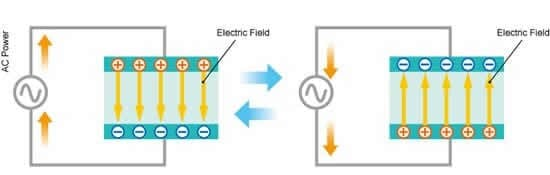Figure 3: Repeatedly charging and discharging

## Coils

A coil, also called an inductor, is yet another primary type of physical component that is used in electronic circuits. It has two leads and is typically implemented as one or more windings (loops) of conductive wire. That wire is often but not necessarily formed around a core of iron or steel or some other magnetic material. Current through the coil induces a magnetic field that serves as a store of energy. Inductance is measured in henries (H).

More specifically, a current flowing through a wire generates a magnetic field, the direction of which is to the right relative to the flow of the current, as described by the 'right-hand rule' (see Figure 4). If the wire is coiled, the fluxes are in alignment. According to Lenz's law, changes in the coil's magnetic field generate a counter-electromotive force (and an induced current) that opposes those changes. Thus, coils can be used in electronic circuits to restrict the flow of alternating current while allowing direct current to pass.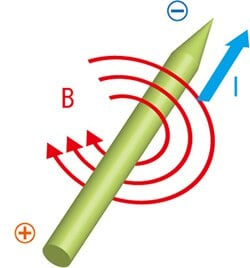Figure 4: Current and magnetic field

Right-Hand Rule:

Current (I) flowing through a conductor produces a magnetic field (B) that circles to the right around the conductor.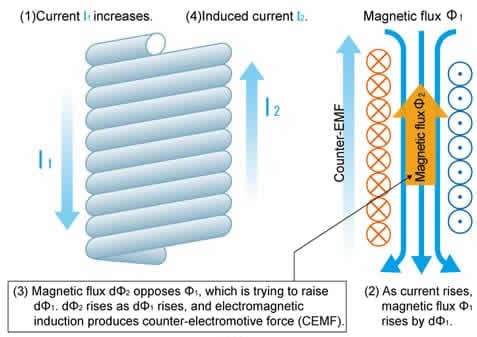Figure 5: Lenz's Law: The induced current in a coil flows in a manner that opposes changes in the number of lines of magnetic force through the coil.

## Filter Circuits (HPFs and LPFs)

A filter circuit is an electrical function made from connected elements that is used to eliminate unwanted electrical signals while allowing wanted signals of specific frequencies to pass. A common type of filter circuit, for example, is an RC-series circuit, in which a resistance and capacitance are connected in series.

RC filters can implement either a high-pass filter (HPF) or a low-pass filter (LPF). An RC filter in which the voltage drop across the resistor (Vr) is taken as the output will pass the high-frequency voltage signals from the input, while filtering out (attenuating) the input's low frequencies (see Figure 6.) By contrast, an RC filter in which the voltage drop across the capacitor (Vc) is taken as the output will allow the low- frequency components of the input signal to get through, but reduce or eliminate the high frequencies (see Figure 7).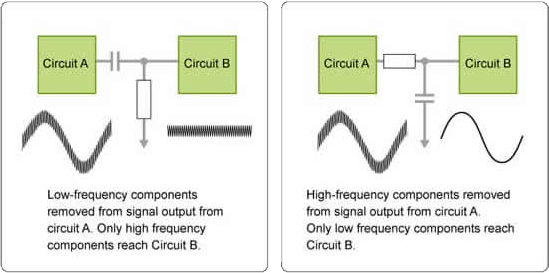Figure 6: High-pass filter (HPF) / Figure 7: Low-pass filter (LPF)

## Module List

1. Passive Elements
2. Diodes, Transistors, and FETs
3. Op-Amps, Comparator Circuit